Example Questions

1 2 81 82 83 84 85 86 87 89 Next →

Example Question #182 : Solid Geometry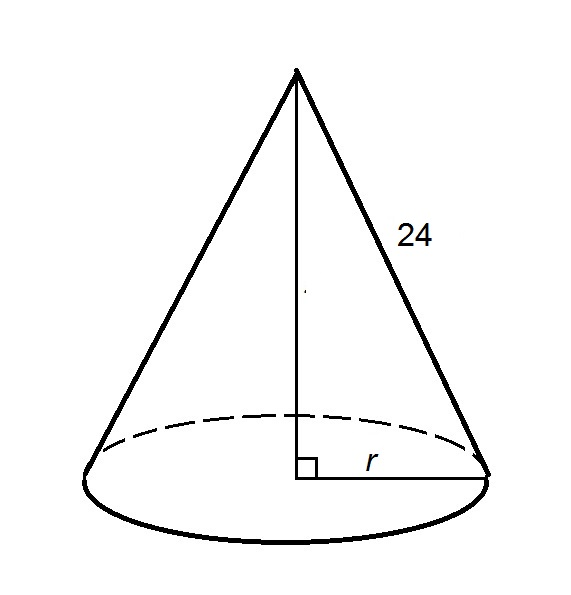In terms of, express the volumeof the above right circular cone.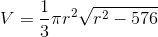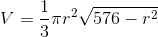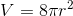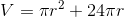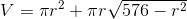Explanation:

The volumeof a cone can be calculated from its heightand the radiusof its base using the formula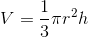The slant heightis shown in the diagram to be 24. By the Pythagorean Theorem,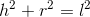Setting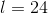and solving for: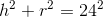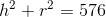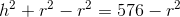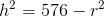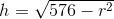Substituting in the volume formula for:.

Example Question #183 : Solid Geometry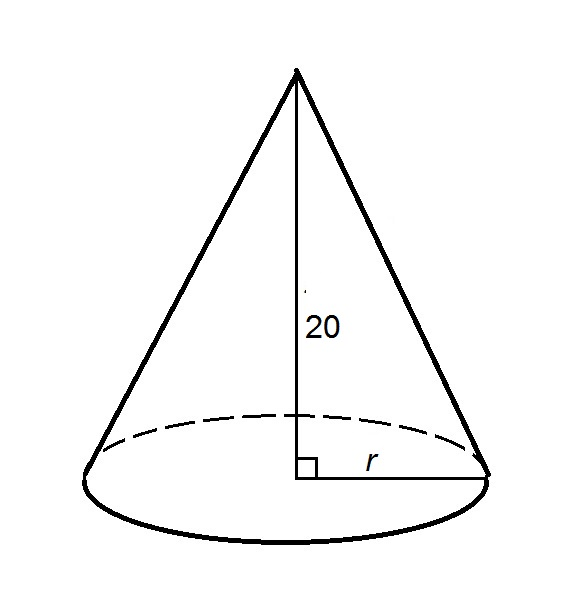In terms of, express the volumeof the provided right circular cone.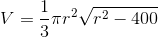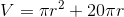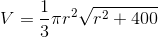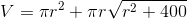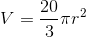Explanation:

The volumeof a cone can be calculated from its heightand the radiusof its base using the formulaThe height of the cone is shown to be equal to 20, so substituting accordingly: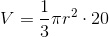1 2 81 82 83 84 85 86 87 89 Next →